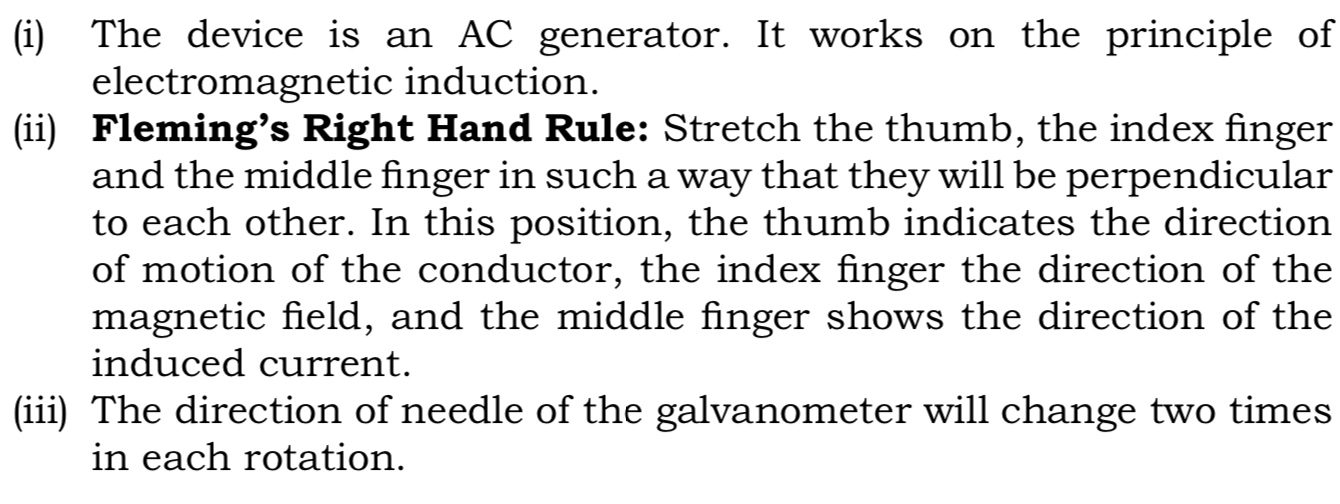SSC BOARD PAPERS IMPORTANT TOPICS COVERED FOR BOARD EXAM 2024

### Science Technology SSC 10th Important Paper for Board Exam 2020.

SCIENCE & TECHNOLOGY - I (72) -
MODEL PAPER NO. 1
IMPORTANT PAPER FOR BOARD EXAM 2020.
SSC MAHARASHTRA BOARD,
10TH STANDARD,

Time : 2 Hours
Max. Marks : 40

Note :
1. It is necessary to solve all the questions.
2. Draw neat and labelled diagrams wherever necessary.
3. Start every new main question on a separate page.
4. Figures on the right indicate marks.
5. For each Multiple Choice Question (1.B), ONLY rst answer will be
considered.
6. Write answer of each MCQ with option number.
Eg. i) a.......... ii) c..............

Q.1. (A) Solve the following questions : 

(1) Fill in the blank :
If objects of equal masses are given equal heat, their nal temperatures will be different, this is due to the difference in their .................. .

Ans. If objects of equal masses are given equal heat, their nal temperatures will be different, this is due to the difference in their specific heat capacity.

(2) Fill in the blank :
Corrosion of copper is called as .................. .

Ans. Corrosion of copper is called as patination.

(3) State whether the following statements are ‘True’ or ‘False’, and explain:
If the separation between two particles is doubled, the gravitational force between the particles becomes half the initial force.

Ans. False - If the separation between two particles is doubled, the gravitational force between the particles becomes one-fourth the initial force.

(4) Fill in the blank :
The capacity of an element to combine is called its .................. .
Ans. The capacity of an element to combine is called its valency.

(5) Consider the rst correlation and then complete the second :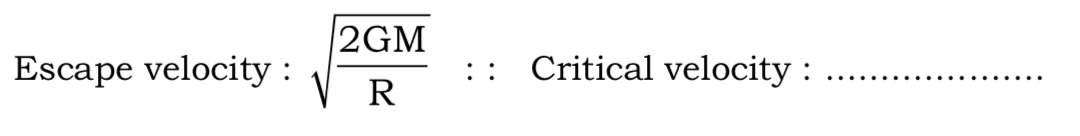Ans.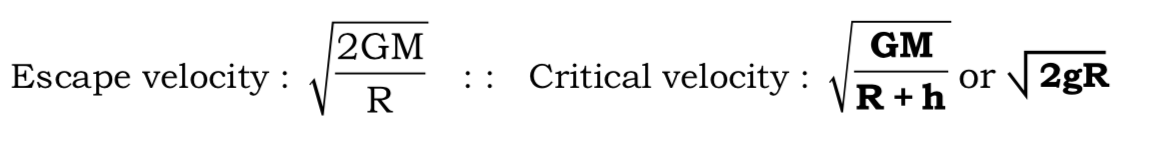Q.1. (B) Choose the correct alternative and rewrite the sentences :
(1)  When Barium chloride solution is added to Sodium sulphate solution, ..................... .
(a) blue precipitate is formed
(b) white precipitate is formed
(c) green precipitate is formed
(d) no reaction takes place

(2)  When a ray of light travels from air to glass and strikes the surface of separation at 90° to the surface, then it ..................... .
(a) bends towards the normal
(b) bends away from the normal
(c) passes without bending
(d) reflects into air

(3)  In Hope’s apparatus when the temperature of water drops from 4°C to 3°C, water .................. .
(a) Remains still
(b) moves upwards
(c) moves downwards
(d) freezes solid

(4)  Due to ..............., vegetable oil is converted into vanaspati ghee.
(a) Hydrogenation
(b) Chlorination
(c) Dehydration
(d) Polymerization

(5)  Rakesh performs the experiment of tracing the path of a ray of light passing through a rectangular glass slab for different angles of incidence. He observes that in all cases ..................... .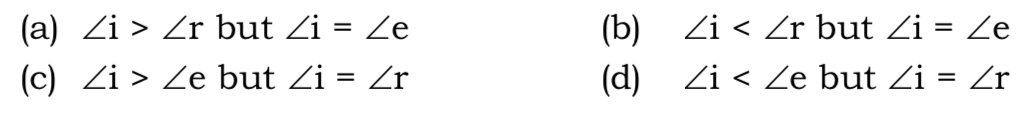Ans. (a)

Q.2. Solve the following questions : (Any 5) 

(1)  Explain what happens when following reaction takes place and give the balanced chemical equation :
Copper reacts with concentrated Nitric acid.
Ans. When copper reacts with concentrated nitric acid, reddish coloured poisonous nitrogen dioxide gas is formed.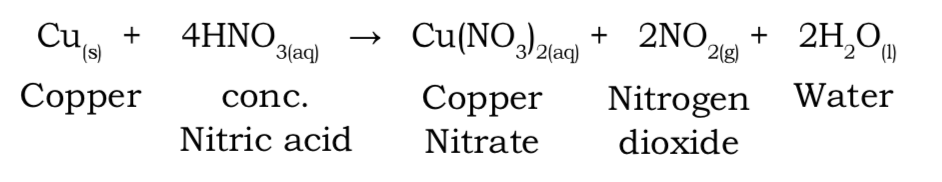(2)  If an artificial satellite is shifted from LEO to HEO, will the value of ‘g’ increase or decrease? Why?
Ans. If an artificial satellite is shifted from LEO to HEO, the value of ‘g’ decreases. This is because as the satellite is shifted from LEO to HEO, its distance from earth’s surface increases. Because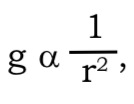as distance increases the value of g will decrease.

(3)  Give Scientific Reason :
Anodes need to be replaced from time to time during the electrolysis of alumina.
Ans. In the hall process of extracting aluminium from aluminium oxide, the electrolyte is a liquid mixture of cryolite (Na3AlF6), fluorspar(CaF2) and aluminium oxide.

(i) The cathode and anodes are made of graphite.

(ii) During the electrolysis process, aluminium is deposited on the cathode on passing electric current.

(iii) Oxygen is liberated at the anode.

(iv) Someofthisoxygenreactswiththecarboninthegraphitetoform carbon - dioxide gas then slowly burning away the anodes.

(v) Thus, the anodes need to be replaced from time to time during the electrolysis of alumina.

(4) Answer the following questions based on the given diagram :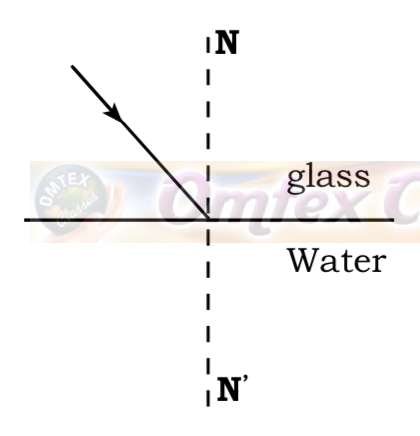(i)  Complete the diagram and state the effect on velocity of light when it enters water.
Ans.When light enters water, its velocity increases.
(ii)  If the light was incident on the interface at an angle greater than the critical angle, which phenomenon occurs?
Ans. Total internal reflection.

(5)  Write structural formula for the following IUPAC name :Butanone
Ans.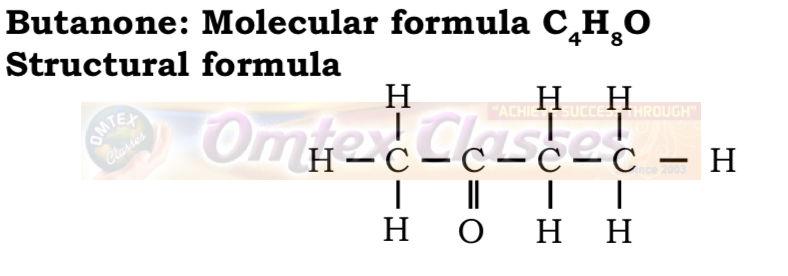(6)  (i) State the law : Modern Periodic Law
Ans. Modern Periodic Law :
Properties of elements are periodic function of their atomic numbers.

(ii) Write the names from the description : Non-metals in the third period.
Ans. Phosphorus, Sulphur, Chlorine and Argon.

(7)  (i) Complete the following diagram :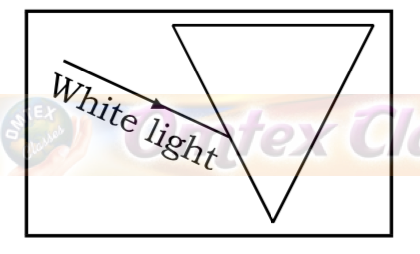Ans.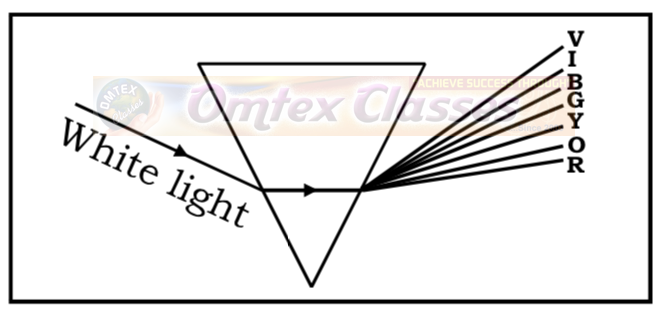(ii) Compare the wavelengths of orange and violet light.
Ans. The wavelength of orange light is more than the wavelength of violet light.

Q.3. Solve the following questions : (Any 5) 
(1) Selwyn is 16 yrs old and wears a spectacle having a power of +2 D. Answer the following questions:
(i) Name the eye defect he is suffering from.
(ii) State two reasons for this defect.
(iii) Compute the focal length of his lens and state the lens used.
Ans. (i) Selwyn is suffering from hypermetropia.
(ii) (a) Curvature of the cornea and the eye lens decreases so that, the converging power of the lens becomes less.
(b) Due to the Flattening of the eyeball the distance between the lens and retina decreases.(2)  How much time, a satellite in an orbit at a height 35780 km above earth’s surface would take to complete one revolution, if the mass of the earth would have been four times its original mass? (consider tangential velocity (V) = 3.08 km/s)
Ans.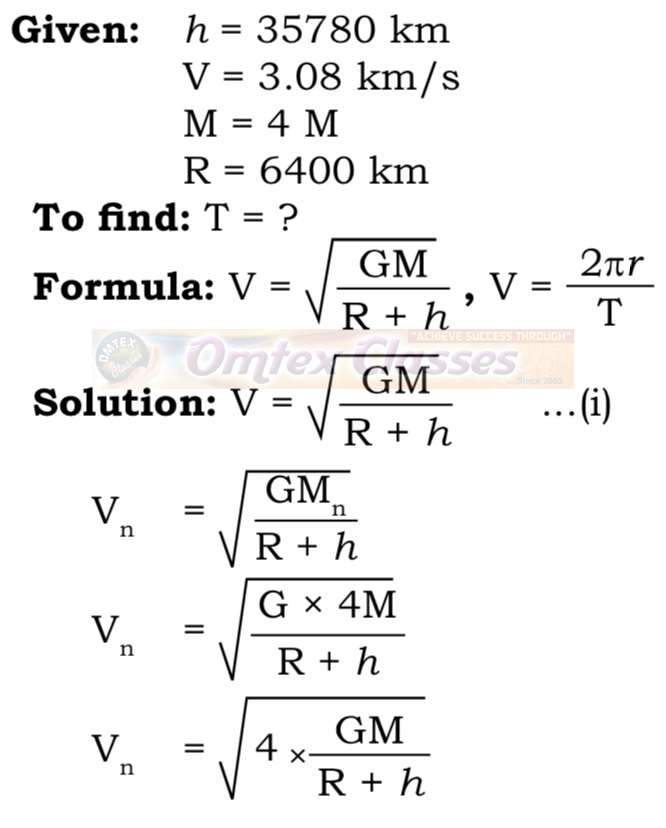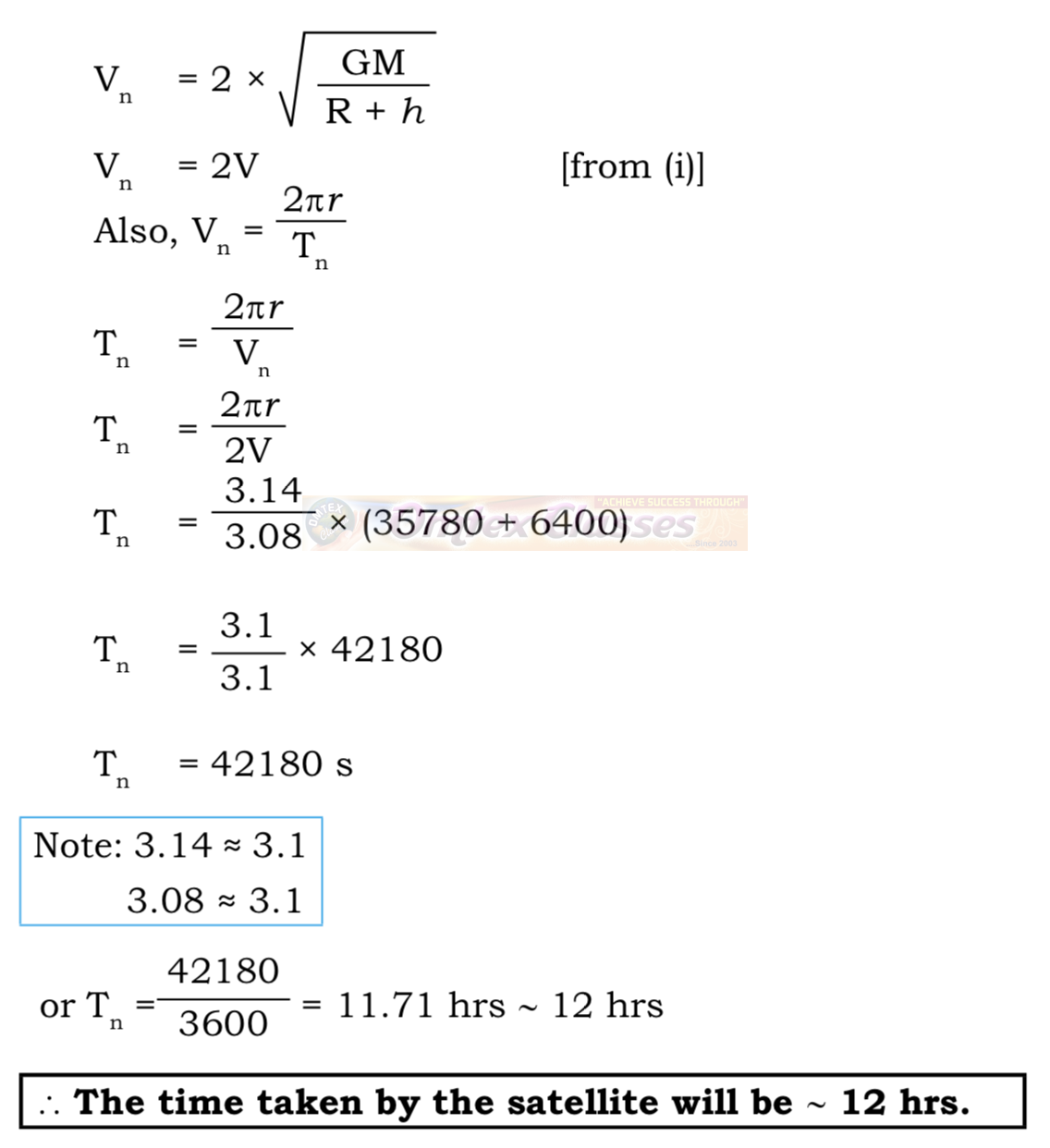(3)  Answer the following based on graph: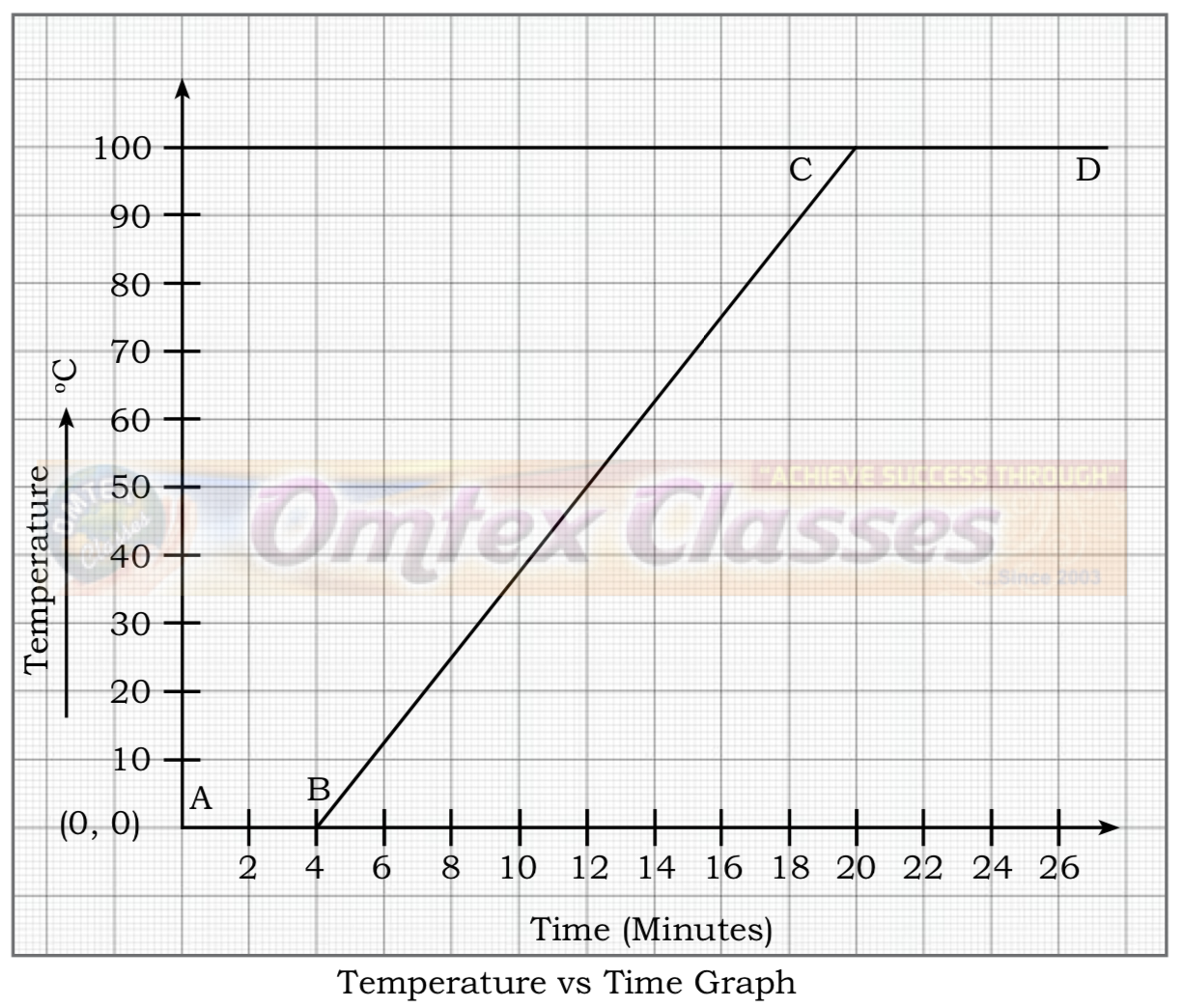(i)  Which parts of the graph show a change of state at constant temperature?
(ii)  Which part of the graph shows a change in temperature without a change in state.
(iii)  Define : Latent heat of Vapourization.
Ans.
(i)  AB and CD

(ii)  BC

(iii) The heat energy absorbed at constant temperature during transformation of liquid into gas is called Latent heat of vapourization.

(4) (i) What are the values of ‘n’ for the shells K, L and M?
(ii) What is the maximum number of electrons that can be accommodated in a shell? Write the formula.
(iii) Deduce the maximum electron capacity of the shells K, L and M.

Ans.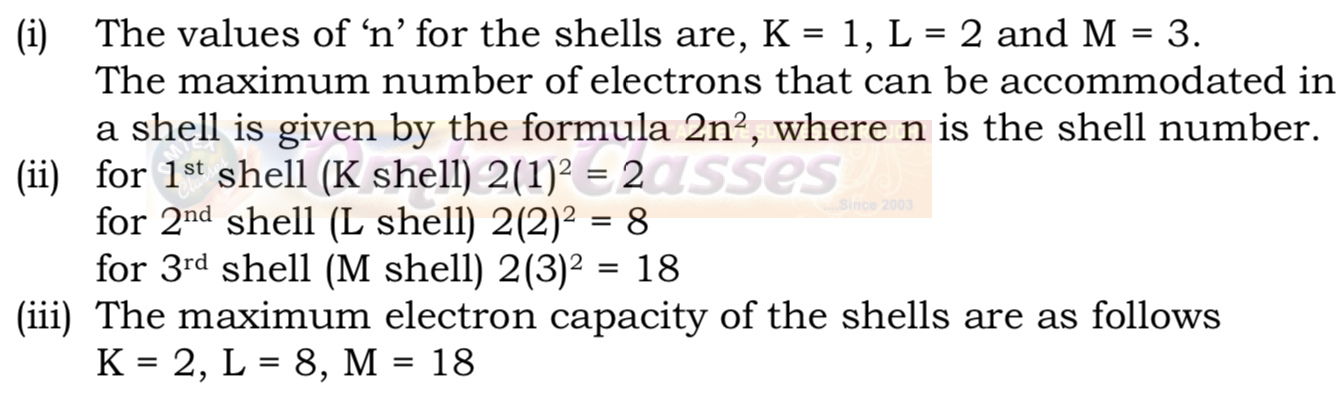(5) Diagram of orbit of a planet moving around the sun.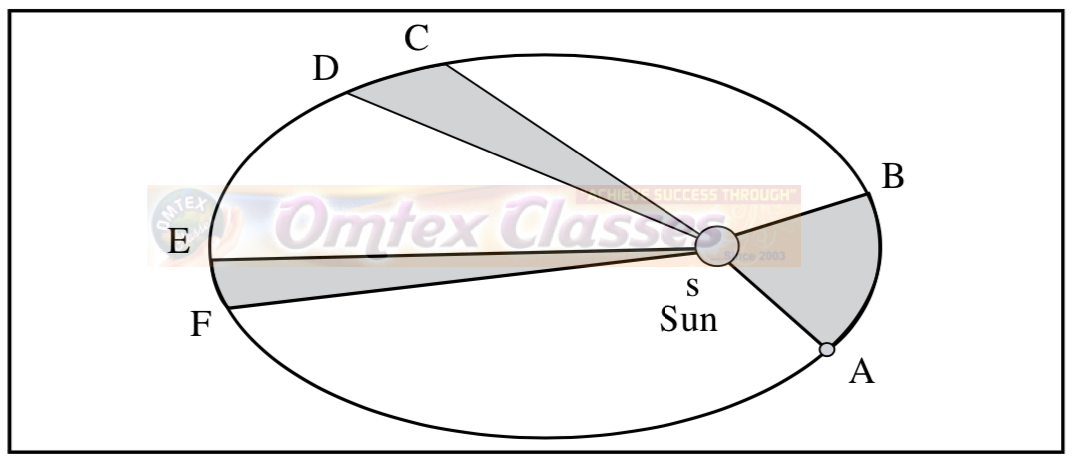(i)  If x, y and z are the speeds of revolution of the planet from A to B, C to D and E to F respectively, then which of the following relation is true?
(a) x > y > z
(b) x < y < z
(c) x < y > z
(d) x > y < z

(ii)  Correct and rewrite Kepler’s third law.

“The period of revolution of a planet around the sun is directly proportional to the cube of the distance of the planet from the sun.’’

Ans. “The square of period of revolution of a planet around the sun
is directly proportional to the cube of the mean distance of the planet from the sun.’’

(6) Observe the following diagram and answer the questions.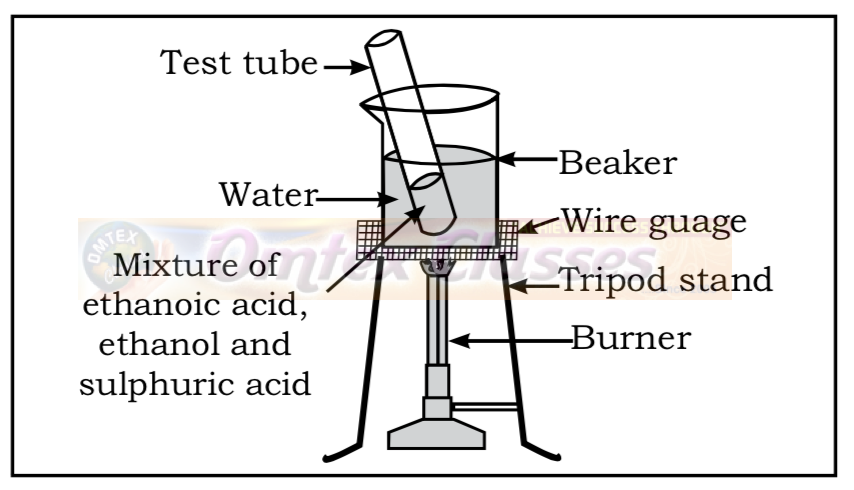(i)  What reaction is shown in the above diagram? Name the reactants and products.
(ii)  Write the chemical equation.
(iii)  What is the special characteristic of the group that is formed in this
reaction?

Ans. (i)  Reaction - Esterification
Reactants - Ethanoic acid and ethanol in presence of concentrated sulphuric acid as catalyst.
Products - Ethyl ethanoate (ester) and water.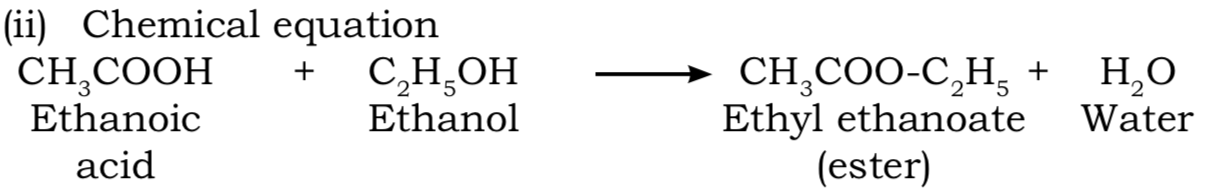(iii) The special characteristic of Esters is that they have sweet odour and hence used for making fragrances and flavouring agents.

(7) What is the reaction called when oxidation and reduction take place simultaneously? Explain with examples.
Ans. When oxidation and reduction take place simultaneously in a given reaction it is termed as Redox reaction. During oxidation a reactant combines with oxygen or loses hydrogen and during reduction it gains hydrogen or loses oxygen.
Example of Redox reaction:Q.4. Solve the following questions : (Any 1)
(1) Redraw the given diagram and label the parts. Complete the paragraph with the words given in the bracket :
(magnetic roller, particles of the magnetic ingredients, away, near, conveyor belt, nonmagnetic part )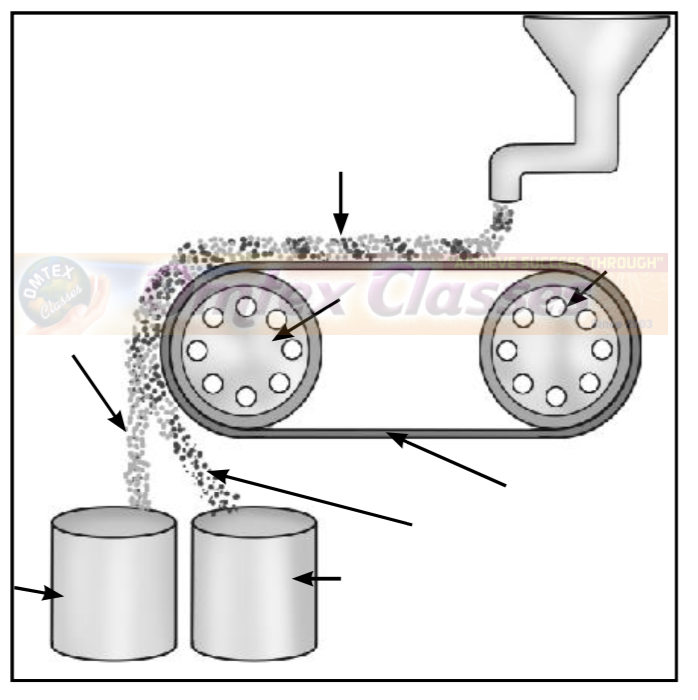The powdered ore is poured on the ____________ near the nonmagnetic roller. Two collector vessels are placed below the magnetic roller.
The particles of the ____________ in the ore are not attracted towards the ____________. Therefore, they are carried further along the belt and fall in the collector vessel placed ____________ from the magnetic roller. At the same time the ____________ of the ore stick to the magnetic roller and therefore fall in the collector vessel ____________ the magnetic roller.

Ans.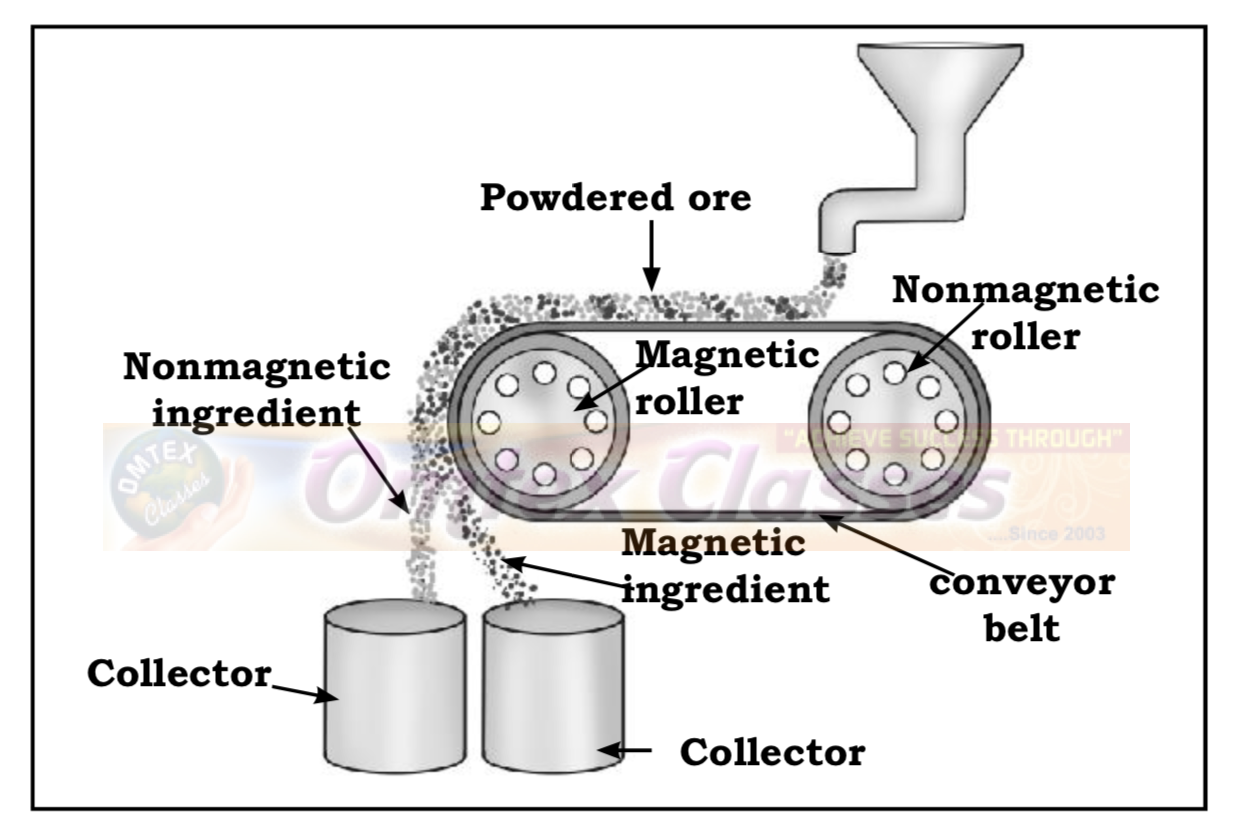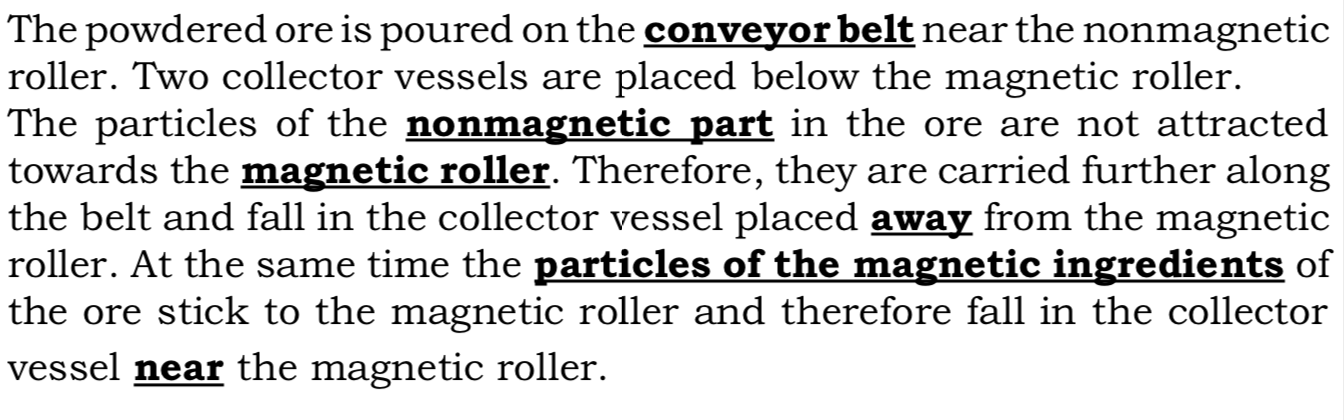(2) From the diagram below answer the following questions :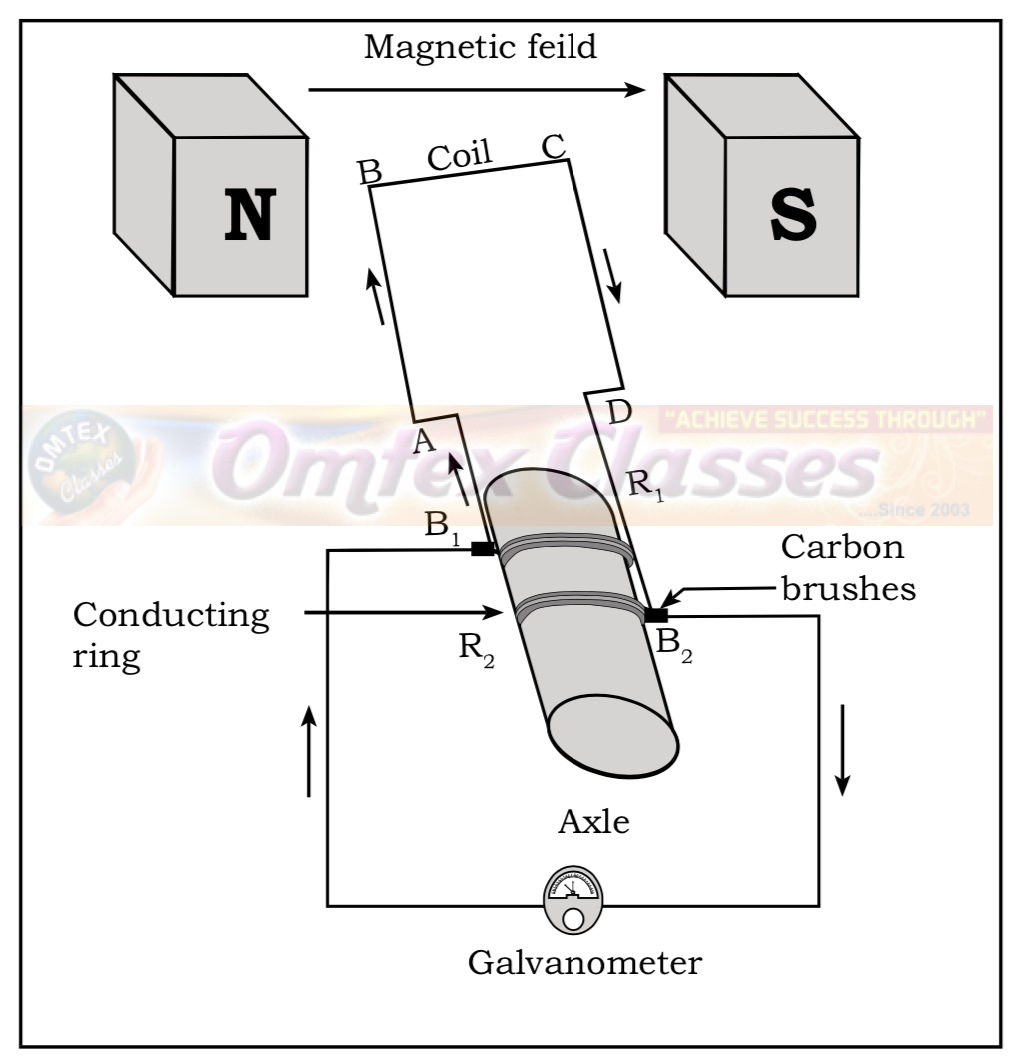(i)  Name the device and the principle on which it works.
(ii)  State the rule that is applicable in its functioning.
(iii) How many times will the direction of needle of the galvanometer change in each rotation?

Ans.